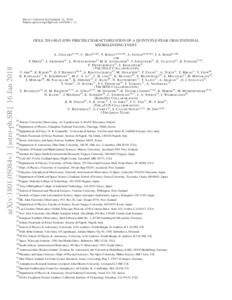# OGLE-2014-BLG-0289: Precise Characterization of a Quintuple-peak Gravitational Microlensing Event

Udalski, A, Han, C, Bozza, V, Gould, A, Bond, IA, Mroz, P, Skowron, J, Wyrzykowski, L, Szymanski, MK, Soszynski, I, Ulaczyk, K, Poleski, R, Pietrukowicz, P, Kozlowski, S, Abe, F, Barry, R, Bennett, DP, Bhattacharya, A, Donachie, M, Evans, P , Fukui, A, Hirao, Y, Itow, Y, Kawasaki, K, Koshimoto, N, Li, MCA, Ling, CH, Masuda, K, Matsubara, Y, Miyazaki, S, Munakata, H, Muraki, Y, Nagakane, M, Ohnishi, K, Ranc, C, Rattenbury, N, Saito, T, Sharan, A, Sullivan, DJ, Sumi, T, Suzuki, D, Tristram, PJ, Yamada, T, Yonehara, A, Bachelet, E, Bramich, DM, DAgo, G, Dominik, M, Jaimes, RF, Horne, K, Hundertmark, M, Kains, N, Menzies, J, Schmidt, R, Snodgrass, C, Steele, IA, Wambsganss, J, Pogge, RW, Jung, YK, Shin, I-G, Yee, JC, Kim, W-T, Beichman, C, Carey, S, Novati, SC and Zhu, W (2018) OGLE-2014-BLG-0289: Precise Characterization of a Quintuple-peak Gravitational Microlensing Event. Astrophysical Journal, 853 (1). ISSN 0004-637XPreview
Text
OGLE-2014-BLG-0289 Precise Characterization of a Quintuple-peak Gravitational Microlensing Event.pdf - Accepted Version

We present the analysis of the binary-microlensing event OGLE-2014-BLG-0289. The event light curve exhibits five very unusual peaks, four of which were produced by caustic crossings and the other by a cusp approach. It is found that the quintuple-peak features of the light curve provide tight constraints on the source trajectory, enabling us to precisely and accurately measure the microlensing parallax ${\pi }_{{\rm{E}}}$. Furthermore, the three resolved caustics allow us to measure the angular Einstein radius ${\theta }_{{\rm{E}}}$. From the combination of ${\pi }_{{\rm{E}}}$ and ${\theta }_{{\rm{E}}}$, the physical lens parameters are uniquely determined. It is found that the lens is a binary composed of two M dwarfs with masses ${M}_{1}=0.52\pm 0.04\ {M}_{\odot }$ and ${M}_{2}=0.42\pm 0.03\ {M}_{\odot }$ separated in projection by ${a}_{\perp }=6.4\pm 0.5\,\mathrm{au}$. The lens is located in the disk with a distance of ${D}_{{\rm{L}}}=3.3\pm 0.3\,\mathrm{kpc}$. The reason for the absence of a lensing signal in the Spitzer data is that the time of observation corresponds to the flat region of the light curve.View Item